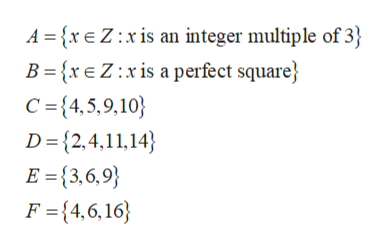# Use the definitions for the sets given below to determine whether each statement is true or false: A = { x ∈ Z: x is an integer multiple of 3 }B = { x ∈ Z: x is a perfect square }C = { 4, 5, 9, 10 }D = { 2, 4, 11, 14 }E = { 3, 6, 9 }F = { 4, 6, 16 }An integer x is a perfect square if there is an integer y such that x = y2.(a)27 ∈ A(b)27 ∈ B(c)100 ∈ B. (d)E ⊆ C or C ⊆ E.(e)E ⊆ A(f)A ⊂ E(g)E ∈ A

Question

Use the definitions for the sets given below to determine whether each statement is true or false:
A = { x ∈ Z: x is an integer multiple of 3 }
B = { x ∈ Z: x is a perfect square }
C = { 4, 5, 9, 10 }
D = { 2, 4, 11, 14 }
E = { 3, 6, 9 }
F = { 4, 6, 16 }
An integer x is a perfect square if there is an integer y such that x = y2.

(a)
27 ∈ A
(b)
27 ∈ B
(c)
100 ∈ B.
(d)
E ⊆ C or C ⊆ E.
(e)
E ⊆ A
(f)
A ⊂ E
(g)
E ∈ A

check_circleExpert Solution
Step 1

From the given information:help_outlineImage TranscriptioncloseA xE Z:xis an integer multiple of 3 B ={xe Z:x is a perfect square C =4,5,9,10 D {2,4,11,14 E{3,6,9 F {4,6,16 fullscreen
Step 2

(a) TRUE

From the definition of set A, elements will be multiple of 3 like:

Step 3

(b) FALSE

From the definition of set B elements will be a perfect square and 27 ...

### Want to see the full answer?

See Solution

#### Want to see this answer and more?

Solutions are written by subject experts who are available 24/7. Questions are typically answered within 1 hour*

See Solution
*Response times may vary by subject and question
Tagged in

### Other